Learning Library
Who we are
Guidance

# 3.NF.A.3 Worksheets, Workbooks, Lesson Plans, and Games

#### CCSS.MATH.CONTENT.3.NF.A.3

:
"Explain equivalence of fractions in special cases, and compare fractions by reasoning about their size."

These worksheets can help students practice this Common Core State Standards skill.

## Worksheets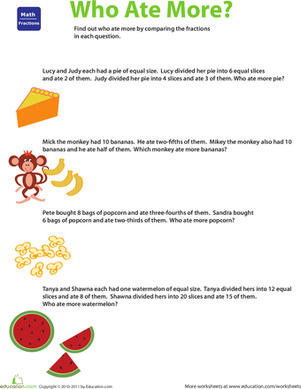Introducing Fractions: Who Ate More?
Worksheet
Introducing Fractions: Who Ate More?
Test the extent of your third grader's fraction knowledge with this fun-to-do worksheet.
Math
Worksheet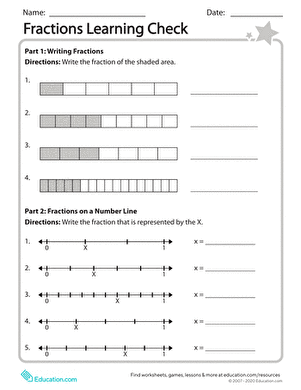Fractions Learning Check
Worksheet
Fractions Learning Check
Use this resource to assess your students’ mastery of concepts surrounding fractions. Your mathematicians will write fractions, find equivalent fractions, compare fractions, and plot fractions on a number line.
Math
Worksheet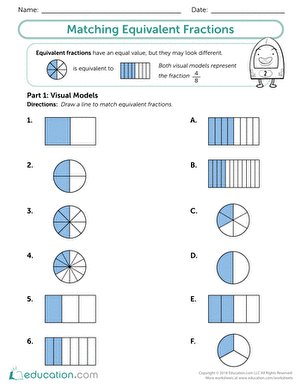Matching Equivalent Fractions
Worksheet
Matching Equivalent Fractions
This matching worksheet is a great way to introduce students to equivalent fractions. This activity will help build your students’ foundational understanding of equivalent fractions with visual models.
Math
Worksheet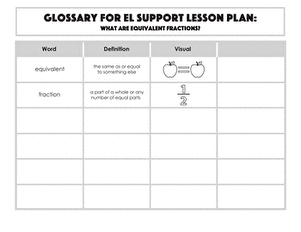Glossary: What Are Equivalent Fractions?
Worksheet
Glossary: What Are Equivalent Fractions?
Use this glossary with the EL Support Lesson: What Are Equivalent Fractions?
Math
Worksheet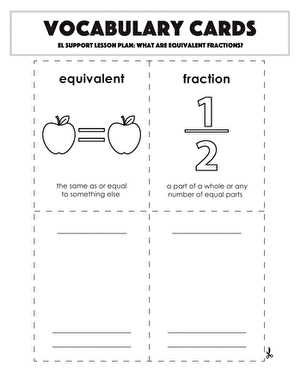Vocabulary Cards:What Are Equivalent Fractions?
Worksheet
Vocabulary Cards:What Are Equivalent Fractions?
Use these vocabulary cards with the EL Support Lesson: What Are Equivalent Fractions?
Math
Worksheet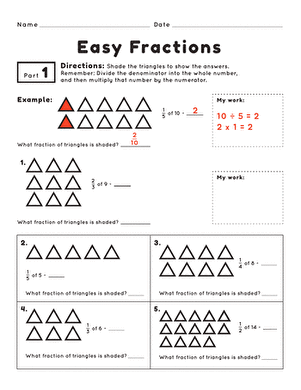Easy Fractions
Worksheet
Easy Fractions
Math
Worksheet

## Lesson Plans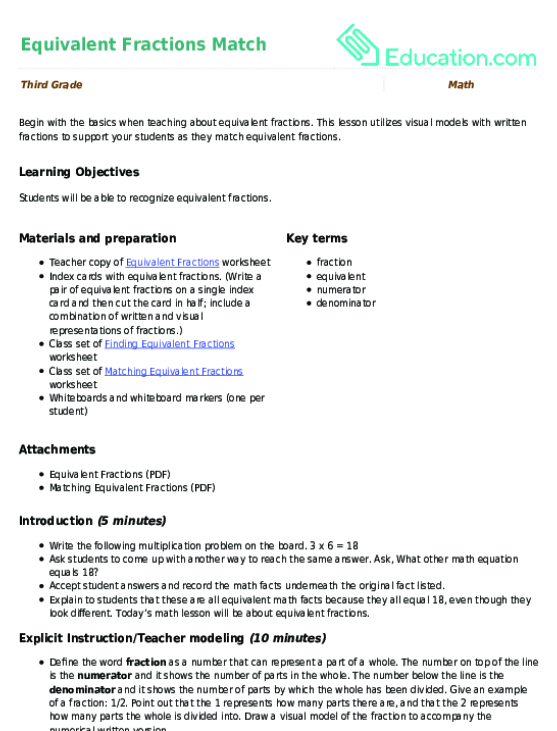Equivalent Fractions Match
Lesson plan
Equivalent Fractions Match
Begin with the basics when teaching about equivalent fractions. This lesson utilizes visual models with written fractions to support your students as they match equivalent fractions.
Math
Lesson plan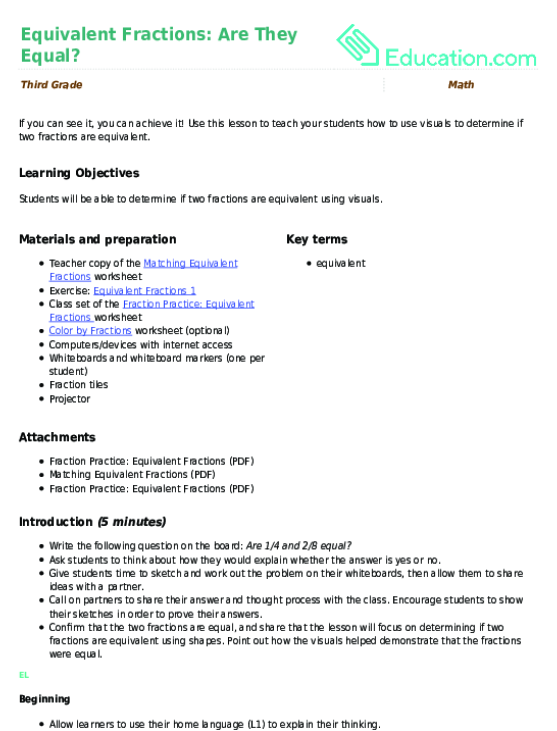Equivalent Fractions: Are They Equal?
Lesson plan
Equivalent Fractions: Are They Equal?
If you can see it, you can achieve it! Use this lesson to teach your students how to use visuals to determine if two fractions are equivalent.
Math
Lesson planWhat Are Equivalent Fractions?
Lesson plan
What Are Equivalent Fractions?
Get your students to think deeply about fractions as they discuss which pictures show equivalence. Use this as a stand alone lesson or a pre-lesson for the *Equivalent Fractions: Are They Equal?* lesson.
Math
Lesson planExplaining Equivalent Fractions
Lesson plan
Explaining Equivalent Fractions
In order to build a strong foundation with fractions, students should be able to explain the concept and their thinking. Use this as a stand alone lesson or as a pre-lesson for *Let's Play Equivalent Fractions!*
Math
Lesson plan

## Workbooks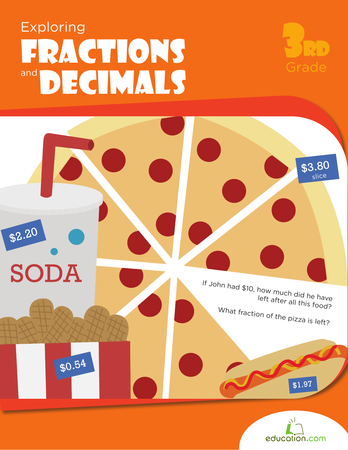Exploring Fractions & Decimals
Workbook
Exploring Fractions & Decimals
Fractions and decimals are way more fun when you apply them to real life scenarios, like parties, food and pets! This workbook teaches kids all about splitting up numbers.
Math
Workbook

## Games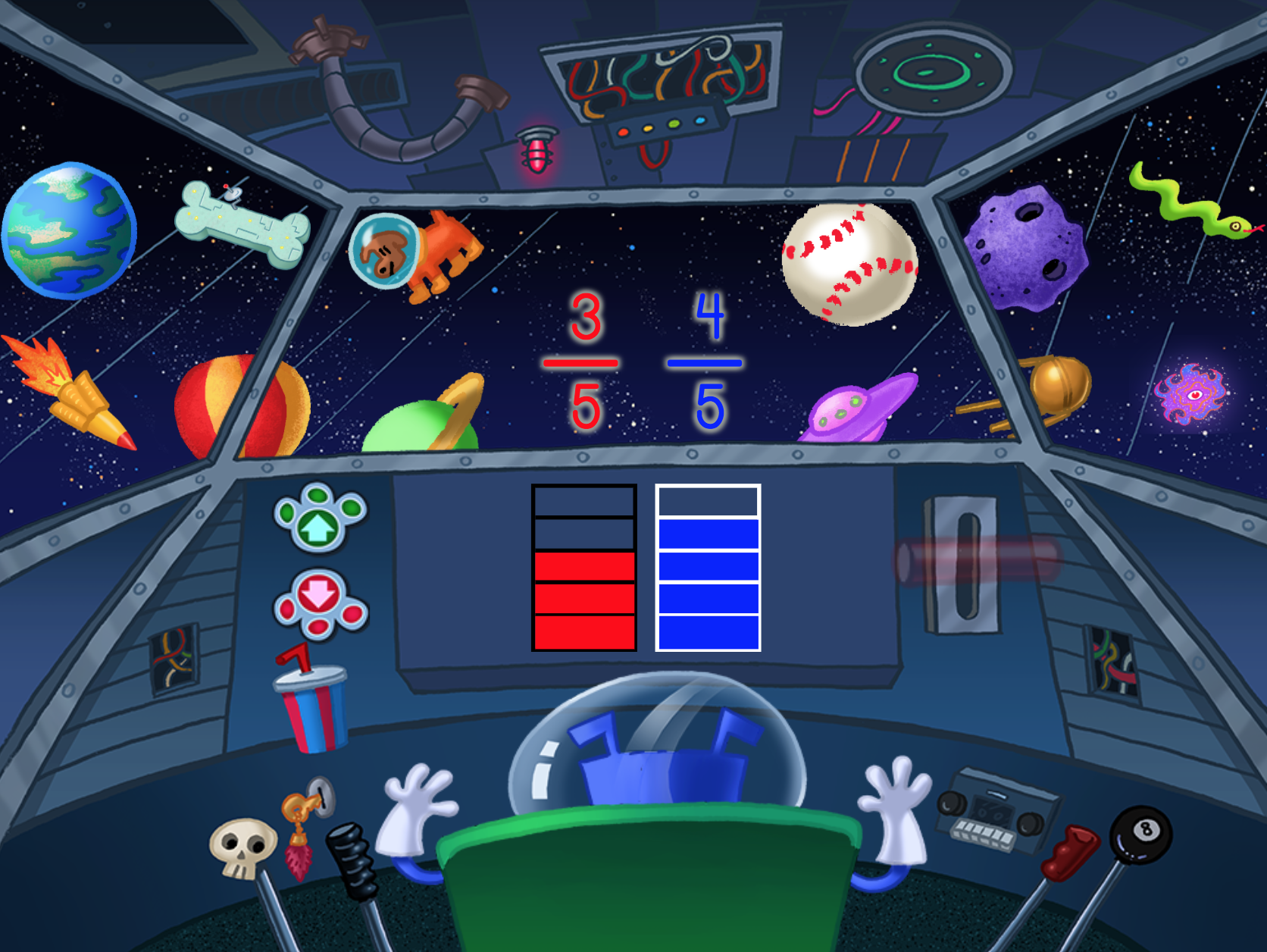Comparing Fractions with Like Denominators: Space Voyage
Game
Comparing Fractions with Like Denominators: Space Voyage
Help your third grader compare fractions with like denominators with this visual area model space game.